# Physical Chemistry : Phases and Properties of Matter

## Example Questions

← Previous 1 3 4

### Example Question #1 : Solids And Liquids

Which of the following is true regarding solids?

None of these

Each solid requires a unique amount of energy to separate the molecules

Solids have high entropy

More than one of these

Each solid requires a unique amount of energy to separate the molecules

Explanation:

There are three main phases: solids, liquids, and gases. Solids molecules are closely packed in an organized, lattice structure, liquid molecules are more spread apart and more disorganized, and gas molecules are spread even farther apart from each other and are extremely disorganized. Each type of solid is made up of unique type of atoms. For example, magnesium metal is made up of magnesium atoms whereas copper metal is made up of copper atoms. Since they are made up of unique atoms, different types of solids have different interactions between atoms. Some have strong interactions whereas others have very weak interactions; therefore, the energy required to break these interaction and separate the atoms/molecules (melt) is different for each solid.

Recall that entropy is the level of disorder in a system. As mentioned, solids are highly organized structures; therefore, they will have the lowest entropy.

### Example Question #31 : Physical Chemistry

You freeze a sample of nitrogen. Compared to the reactant, the end product has __________ density and __________ mass.

a higher . . . the same

the same . . . the same

a higher . . . a higher

a lower . . . the same

a higher . . . the same

Explanation:

Freezing the is process of converting a liquid to a solid. This question is asking about the freezing process of liquid nitrogen to solid nitrogen; therefore, the end product of the reaction is solid nitrogen. Recall that solids are more tightly packed. This means that the volume taken up by the molecules in solid is lower than in liquid; therefore, solids generally have a lower volume. Mass, on the other hand, depends on the number of molecules present. Phase changes do not alter the amount of molecules present. For example, the end product in this question (solid nitrogen) will have the same amount of molecules as its liquid counterpart; therefore, the mass doesn’t change when the phase changes.

Density is defined as follows.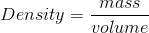Since its volume decreases and the mass stays the same, a solid will have a lower density than liquid. Note that water is an exception to this general rule, as solid ice has a lower density (higher volume) than the same mass of liquid water. This is due to the arrangement of its hydrogen bonds throughout its crystalline structure.

### Example Question #1 : Properties Of Liquids

An unknown molecule (molecule A), in its solid phase, is found to have a density of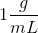. Eight grams of this molecule is added to a cubic container with length of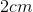. The container is then heated until all of the solid has melted. What can you conclude from the given information?

Liquid will overflow

Solid will not fit in the container

The container will be about halfway full with liquid

No liquid will overflow

Liquid will overflow

Explanation: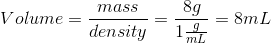The dimensions of the cubic container arebyby(cube has same length, height, and width); therefore, the volume of the cubic container is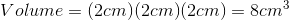Recall that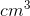is the same as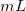; therefore, the container can contain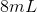of volume. This means that all of the solid will fit into the container. Upon melting, the solid will expand and the volume will increase (as it becomes liquid). This means that the volume of the container will not be sufficient for the liquid and, consequently, lead to an overflow of the liquid.

### Example Question #1 : Phases And Properties Of Matter

Which of the following is true regarding the liquid phase?

I. Liquids have lower entropy than gases

II. Compared to solids, more energy is required to separate liquid molecules

III. Liquids have higher intermolecular forces than gases

III only

I, II and III

I and II

I only

I, II and III

Explanation:

Liquid is an intermediate phase, between solid and gas. Solids are characterized by tightly packed molecules in an organized manner whereas gases are characterized by greatly spread out molecules that are highly disordered; liquids lie somewhere in the middle. This means that they are more disordered than solids (more entropy) and less disordered than gases (less entropy).

The boiling point of a substance is always higher than the melting point; therefore, you always need higher energy to break the interactions between liquid molecules.

The key difference between a liquid and a gas is the distance between the molecules. Liquid molecules are closer together whereas gas molecules are spread apart. Intermolecular forces are the forces between adjacent molecules. Since they are highly spread apart, the gas molecules have lower intermolecular forces.

### Example Question #1 : Solids And Liquids

Which of the following are responsible for the high boiling point of water, compared to molecules of similar size?

Two lone pairs of electrons

Dipole-dipole interactions

Hydrogen bonding

Positive charge

Molecular size

Hydrogen bonding

Explanation:

Hydrogen bonding occurs between water molecules. While intermolecular forces between molecules exist for most molecules, these forces are much weaker than the bond formed between a hydrogen from one molecule and an oxygen, fluorine, and/or nitrogen atom of another molecule. Since the molecules are held more tightly together, more energy is required to break those bonds. This results in a higher temperature required to boil water (boiling breaks bonds between molecules, and this causes the molecules to escape into the gas phase).

### Example Question #1 : Phases And Properties Of Matter

An object is floating on water. Which of the following is true?

I. The object has a lower density than water

II. The buoyancy force would be higher if the object sank

III. The volume of fluid displaced would be higher if the object sank

II only

II and III

I and III

I and II

I and III

Explanation:

Recall than an object floats on water if it has a lower density than water. A floating or submerged object in a liquid has a gravitational pull (downward force) equal to the buoyancy force (upward force). The force due to gravity (gravitational pull) depends only on the mass of the object. In this case, the object can never be submerged because the density of the solid cannot be changed (unless the phase of the object is changed). To submerge, the object has to be placed in a liquid with LOWER density.

The volume of fluid displaced depends on the volume occupied by the object in the liquid. When an object floats, only part of the object is inside the liquid. This means that only part of the object’s volume is occupied inside the liquid (lower volume of fluid displaced). If the object were to be submerged (in a different liquid), the entire volume of the object would be inside the liquid and there would be an increase in the volume of fluid displaced.

### Example Question #1 : Phases And Properties Of Matter

Fluid A and Fluid B flow through the same pipe. However, it is found that fluid A flows much slower than fluid B. What can you conclude about fluid A and fluid B?

None of these

Both of these

The forces between layers in Fluid A is lower

Fluid A has a higher coefficient of viscosity

Fluid A has a higher coefficient of viscosity

Explanation:

Viscosity is a measure of resistance to flow. A liquid with high viscosity is said to have strong forces between its layers, making it harder to flow whereas a liquid with low viscosity has weak forces between its layers, making it easier to flow. Viscosity is quantified by the coefficient of viscosity (higher the coefficient, higher the viscosity and higher the resistance to flow). The question states that fluid A has more trouble flowing; therefore, fluid A has higher coefficient of viscosity and stronger forces between layers.

### Example Question #1 : Solids And Liquids

According to Poiseuille’s principle, the flow rate __________ as viscosity increases and the flow rate __________ as length of pipe increases.

decreases . . . decreases

decreases . . . increases

increases . . . increases

increases . . . decreases

decreases . . . decreases

Explanation:

Poiseuille’s principle quantifies the flow rate of a fluid through a pipe to numerous variables as follows.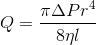where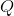is the flow rate,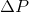is change in pressure,is radius,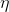is viscosity, andis length. We can see that viscosity and length terms are in the denominator; therefore, flow rate and these two terms are inversely related. This means that increasing viscosity and/or length of pipe will decrease the flow rate (and vice versa). Note that the radius has the biggest exponential factor; therefore, altering radius of the pipe will contribute to the biggest change in flow rate.

### Example Question #1 : Viscosity And Flow Mechanics

One end of a pipe (End A) has twice the radius as the second end (End B). What can you conclude about the flow of fluid through this pipe?

Fluid will flow faster and have a higher pressure at End B

Fluid will flow faster and have a lower pressure at End A

Fluid will flow faster and have a lower pressure at End B

Fluid will flow faster and have a higher pressure at End A

Fluid will flow faster and have a lower pressure at End B

Explanation:

To solve this question, we need to use the continuity equation and Bernoulli’s equation. The continuity equation is as follows: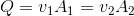whereis flow rate,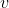is velocity, andis the area. This equation states that that the flow rate of a fluid is the same, regardless of changes in velocity and area. The equation implies that as the area is increased (for example,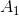) the velocity (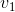) decreases to maintain a constant flow rate and vice versa. The question states that End A has the larger radius; therefore, the velocity is lower at End A.

Bernoulli’s equation is as follows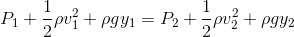whereis pressure,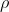is density,is velocity,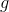is acceleration due to gravity, andis the height from ground. This equation implies that as velocity is increased on one side, the pressure decreases to compensate. Since we already determined that End B has the higher velocity, it will also have the lower pressure.

### Example Question #2 : Phases And Properties Of Matter

Why is liquid water more dense than ice?

None of these

Liquid water and solid ice have the same density

The slope of the solid/liquid phase line for water is positive

Water crystalizes with greater average space between molecules

Water has metastable phases